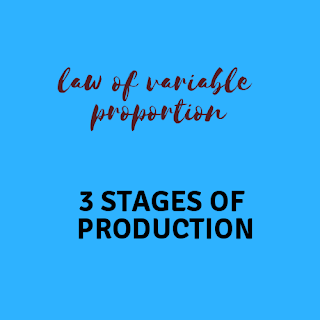# Economics Articles Library

Articles related with Micro Economics, Macro economics Accountancy and Business studies useful for XI, XII, CS foundation, CA(CPT), BBA , B.com,M.com and for other readers.

## Sunday, 2 September 2018

Law of variable proportions

This law is related to the short run production function in which output is increased only by increased application of variable factor. Students and reader must note that “ labour” is considered variable factor for this purpose.

This law states about the behaviour of output when we continuously increase variable factor. Fixed factor remains constant in this case.

According to the law of variable proportions when we increase variable factor continuously then following results are obtained:Law of variable proportions

# stage 1

Total product increases with increasing rate. Increasing rate means additions in total product increases. see the table below for better understanding.

# stage 2

Total product increases with decreasing rate. See the table below.

# stage 3

Total product starts to decrease.

This behaviour of total product is based on marginal product. Let's talk about marginal product behaviour in all three stages.

# stage 1

Marginal product increases.

# stage 2

Marginal product decreases

# Stage 3

Negative marginal product.

Table-. Law of variable proportions

Explanation to the table:

In the above table till the variable factor 3 , producer is experiencing 1st stage. TP is increasing at increasing rate. This stage is known as increasing returns to factor.

Till the variable factor 4 and 5 , TP is increasing at decreasing rate. This is 2nd stage and known as decreasing returns to factor.

At the variable factor 6, TP becomes maximum. Eventually a situation comes when TP starts to decrease. This is 3rd stage known as negative returns to factor.

Assumptions

• Law of variable proportion operates only in short run.
• Variable factors are homogeneous
• Technology is constant

If above assumptions disturb then law operates to fail. This is also called as postponement of the law.

Where will producer stop ?

Producer will stop himself to produce when he will get constant returns to factor. This situation will arise somewhere between stage 2 and stage 3.

Important Definitions

According to F.Benham

“As the proportion of one factor in a combination of factors is increased, after a point, first the marginal and then the average product of that factor will diminish.”

An increase in some inputs relative to other fixed inputs will, in a given state of technology, cause output to increase; but after a point the extra output resulting from the same addition of extra inputs will become less.” (Paul A. Samuelson)

1.2.